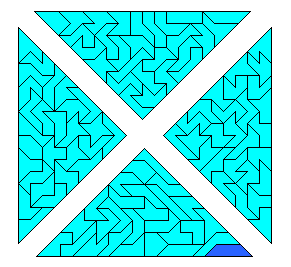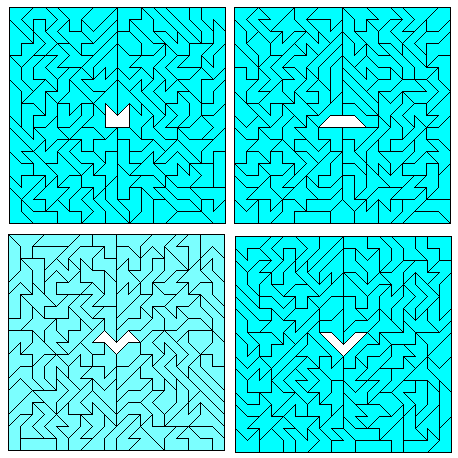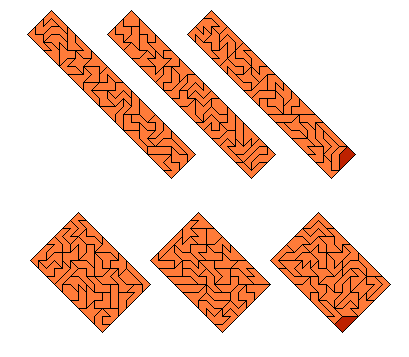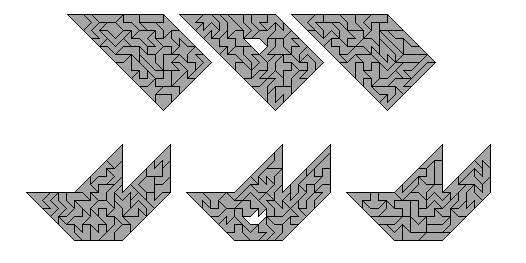## Using One Piece Twice

If we are allowed to use one hexabolo twice, we get 108 pieces with a total area of 316. This number factorises very well and a lot of constructions are possible.
Four isosceles rectangular triangles are versatile as they can be combined into a 18s x 18s square, two 9h x 9h squares, a large triangle and a parallelogram.If we want the additional piece to be placed in the center of a 18s x 18s square a special construction is necessary. For the "house" as the additional piece a solution is shown on the Poly Pages . There are only five symmetric pieces, which are balanced and have odd slope and therefore meet the the constraints for such a construction. The solutions for the four remaining pieces are shown here.The cross is another nice shape to put the additional piece in the center of the construction.Let's have a look at the rectangles. With the two 27s x 6s or the three 18s x 6s rectangles you can get the 54s x 6s and the 27s x 12s rectangles, too. The two 18s x 9s or the three 12s x 9s rectangles can be combined into the 36s x 9s. The four 9s x 9s squares seem to be more difficult and I haven't got a solution yet.Besides the two 9h x 9h squares, which can be derived form the four triangles, two sets of multiple h-rectangles are shown: three 18h x 3h and three 9h x 6h rectangles, which combine into a 54h x 3h and a 27h x 6h respectively.With 108=3*36 three 6-fold replicas of all pieces might be possible. It would be nice to use the replicated piece twice, but due to the constraints this can only be done for the balanced pieces with odd slope. For the five symmetric pieces meeting this condition the solutions are shown.I think a solution for the other pieces is also possible since I have found the replicas even for a piece with three acute cornersAmong the pieces which need a different additional piece to get replicated there are some hard examples: the star with four acute corners and pieces with a boundary of 8 s-sides. For the "bar" three 18s x 6s rectangles are the solution as seen above.index - previous - next